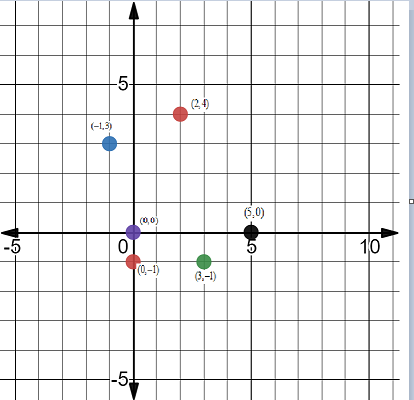Chapter 0.D, Problem 1DT### Algebra and Trigonometry (MindTap ...

4th Edition
James Stewart + 2 others
ISBN: 9781305071742

#### Solutions

Chapter
Section### Algebra and Trigonometry (MindTap ...

4th Edition
James Stewart + 2 others
ISBN: 9781305071742
Textbook Problem
1 views

# D DIAGNOSTIC TEST: The Coordinate PlaneGraph the following points in a coordinate plane. ( a )   ( 2 , 4 ) ( b )   ( − 1 , 3 ) ( c )   ( 3 , − 1 ) ( d )   ( 0 , 0 ) ( e )   ( 5 , 0 ) ( f )   ( 0 , − 1 )

To determine

To draw:

The graph by using the given coordinate (2,4), (1,3), (3,1), (0,0), (5,0), (0,1).

Solution:Explanation

Calculation:

Given: Coordinates are,

(2,4), (1,3), (3,1), (0,0), (5,0), (0,1) have to plot this points in the graph.Therefore the points are plotted.

#### The Solution to Your Study Problems

Bartleby provides explanations to thousands of textbook problems written by our experts, many with advanced degrees!

Get Started